How do you do #18 in Test 6 Section 3 without a calculator?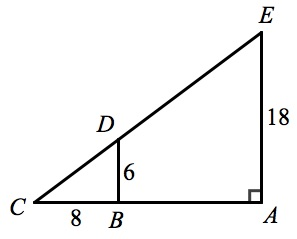The key to this one is recognizing that you’ve got similar triangles. First, both triangle CDB and triangle CEA contain angle C. The question also tells you that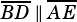, which means that angle CDB is also a right angle. Therefore, you have angle-angle similarity.

It’s also good to recognize a 6-8-10 triangle quickly. Know your Pythagorean triples! 🙂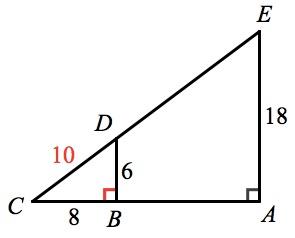From there, all you need to do is recognize that the length ofis 3 times the length of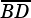, which means all the sides of triangle CEA are 3 times the length of their corresponding sides in triangle CDB. Therefore, because the length of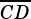is 10, the length ofmust be 30.